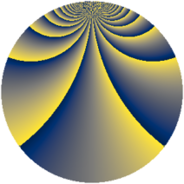# Properties

 Label 2880.2.zLevel $2880$ Weight $2$ Character orbit 2880.z Rep. character $\chi_{2880}(847,\cdot)$ Character field $\Q(\zeta_{4})$ Dimension $116$ Sturm bound $1152$

# Learn more about

## Defining parameters

 Level: $$N$$ $$=$$ $$2880 = 2^{6} \cdot 3^{2} \cdot 5$$ Weight: $$k$$ $$=$$ $$2$$ Character orbit: $$[\chi]$$ $$=$$ 2880.z (of order $$4$$ and degree $$2$$) Character conductor: $$\operatorname{cond}(\chi)$$ $$=$$ $$80$$ Character field: $$\Q(i)$$ Sturm bound: $$1152$$

## Dimensions

The following table gives the dimensions of various subspaces of $$M_{2}(2880, [\chi])$$.

Total New Old
Modular forms 1216 124 1092
Cusp forms 1088 116 972
Eisenstein series 128 8 120

## Trace form

 $$116q + 2q^{5} + 4q^{7} + O(q^{10})$$ $$116q + 2q^{5} + 4q^{7} - 4q^{11} + 4q^{17} - 8q^{19} - 4q^{23} - 4q^{35} - 24q^{47} + 4q^{53} + 4q^{55} - 16q^{59} - 20q^{61} + 4q^{65} - 40q^{71} - 8q^{73} + 32q^{77} - 44q^{83} + 8q^{85} - 12q^{91} - 40q^{95} - 4q^{97} + O(q^{100})$$

## Decomposition of $$S_{2}^{\mathrm{new}}(2880, [\chi])$$ into newform subspaces

The newforms in this space have not yet been added to the LMFDB.

## Decomposition of $$S_{2}^{\mathrm{old}}(2880, [\chi])$$ into lower level spaces

$$S_{2}^{\mathrm{old}}(2880, [\chi]) \cong$$ $$S_{2}^{\mathrm{new}}(80, [\chi])$$$$^{\oplus 9}$$$$\oplus$$$$S_{2}^{\mathrm{new}}(240, [\chi])$$$$^{\oplus 6}$$$$\oplus$$$$S_{2}^{\mathrm{new}}(320, [\chi])$$$$^{\oplus 3}$$$$\oplus$$$$S_{2}^{\mathrm{new}}(720, [\chi])$$$$^{\oplus 3}$$$$\oplus$$$$S_{2}^{\mathrm{new}}(960, [\chi])$$$$^{\oplus 2}$$